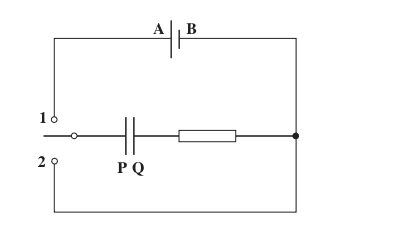# Capacitors -- charging and discharging

Hannah7h
So, is this true for when a capacitor charges through a fixed resistor (as shown in the image below, when the switch is closed to 1)- the potential difference across the resistor exponentially decreases to zero and the potential difference across the capacitor exponentially increases from zero to equal the voltage across the battery (power supply), when the capacitor is fully charged?Homework Helper
Gold Member
So, is this true for when a capacitor charges through a fixed resistor (as shown in the image below, when the switch is closed to 1)- the potential difference across the resistor exponentially decreases to zero and the potential difference across the capacitor exponentially increases from zero to equal the voltage across the battery (power supply), when the capacitor is fully charged?
View attachment 101750
Yes.

Hannah7h
Yes.

Ok, so now if the switch is moved to position 2 and the capacitor is now discharging. The potential difference of the capacitor will now decrease exponentially, and so does the potential difference across the fixed resistor- why does the p.d across the fixed resistor also decrease?

David Lewis
Also, when switch is in position 1, you could say voltage across the cap rises asymptotically to battery voltage.

When switch is in position 2, you can then think of the capacitor as a power supply -- where the voltage drops as you draw current out of it.

Homework Helper
Gold Member
Ok, so now if the switch is moved to position 2 and the capacitor is now discharging. The potential difference of the capacitor will now decrease exponentially, and so does the potential difference across the fixed resistor- why does the p.d across the fixed resistor also decrease?
Capacitor acts as a source while discharging and voltage across capacitor is equal to the voltage across resistor. Since capacitor voltage is decreasing, voltage across the fixed resistor is also decreasing.

Homework Helper
Gold Member
In other words, resistor does not store any charge. Hence, voltage across the resistor decreases while discharging of the capacitor.

Hannah7h
Capacitor acts as a source while discharging and voltage across capacitor is equal to the voltage across resistor. Since capacitor voltage is decreasing, voltage across the fixed resistor is also decreasing.

Ohhh I see that makes sense, just one more question why in: VC= -VR is the p.d across the resistor quoted as a negative value?

Hannah7h
Also, when switch is in position 1, you could say voltage across the cap rises asymptotically to battery voltage.

When switch is in position 2, you can then think of the capacitor as a power supply -- where the voltage drops as you draw current out of it.

Ah ok yes this makes sense now

Homework Helper
Gold Member
Ohhh I see that makes sense, just one more question why in: VC= -VR is the p.d across the resistor quoted as a negative value?
It follows from KVL i.e. Vc+Vr=0. Going along the direction of current, you'd see a drop in potential across the resistor and a gain of potential across the capacitor.

Hannah7h
It follows from KVL i.e. Vc+Vr=0. Going along the direction of current, you'd see a drop in potential across the resistor and a gain of potential across the capacitor.

Ok yeah makes sense, so when the capacitor is discharging the p.d across the capacitor increases so the p.d across the resistor decreases in order to = zero. And then when the capacitor discharges VC= -VR as both the p.d across the capacitor and p.d across the resistor decrease to zero.

Homework Helper
Gold Member
Ok yeah makes sense, so when the capacitor is discharging **charging** the p.d across the capacitor increases so the p.d across the resistor decreases in order to = zero
And then when the capacitor discharges VC= -VR as both the p.d across the capacitor and p.d across the resistor decrease to zero.
Right.

Hannah7h
Right.

Sorry my mistake! and ok good, thanks for the help!

Homework Helper
Gold Member
Sorry my mistake! and ok good, thanks for the help!
You're welcome!

lychette
Also, when switch is in position 1, you could say voltage across the cap rises asymptotically to battery voltage.

When switch is in position 2, you can then think of the capacitor as a power supply -- where the voltage drops as you draw current out of it.

Rises exponentially is the correct and precise term!
•